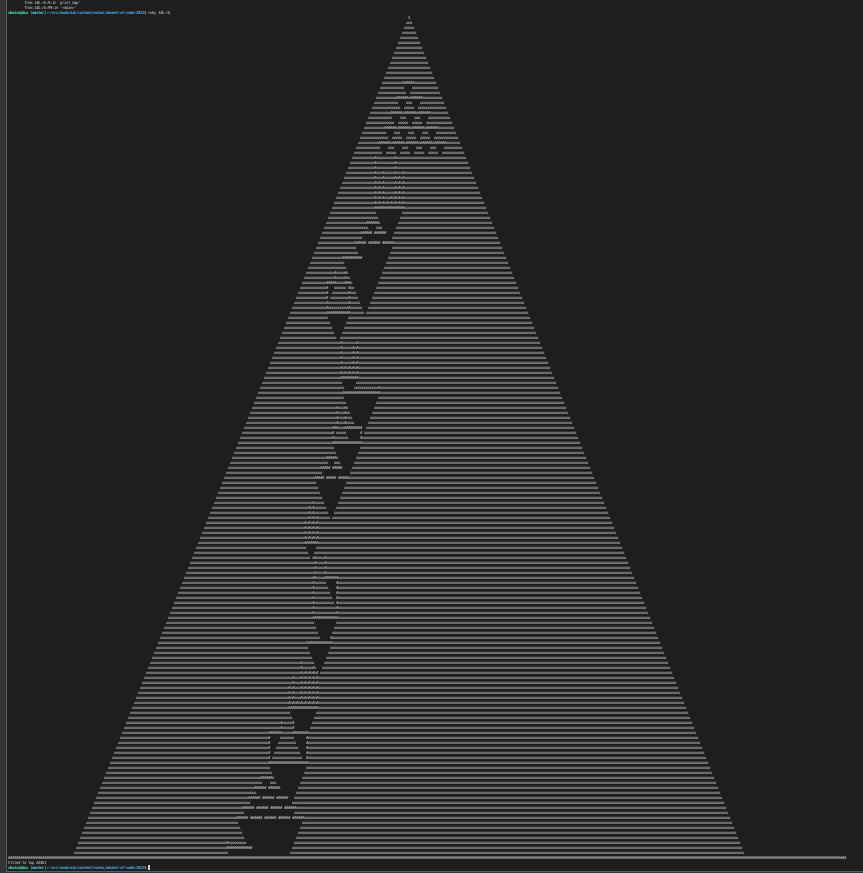# 14 Ruby

I’ve programmed in Ruby years ago (an online language annotation tool). Not much of Ruby has remained in my brain since. :)

## Part I Assignment

``````# init large enough 2D array, fill with spaces
map = Array.new(1000){Array.new(1000," ")}
maxcol = 0
maxrow = 0

# printing of the map with sand for debugging
def print_map(map, maxrow, maxcol)
for i in 0..maxrow do
# print only the interesting part of map
for j in 300..maxcol do
# sand source
if j == 500 and i == 0
print "X"
# rock
elsif map[i][j] == '#'
print map[i][j]
# sand
elsif map[i][j] == 'o'
print map[i][j]
# empty space
else
print " "
end
end
# print doesn't print newline by default
print "\n"
end
end

# parse input and obtain the map
# remember the last coordinates
# within a line
# so we can draw lines between pairs
prev_row = nil
prev_col = nil
# split by spaces and process tokens
line.split(" ").each do |token|
if token.include? ","
# num1,num2 => split into two integers
col_s, row_s = token.split(",")
col = col_s.to_i # string to integer
row = row_s.to_i
# search for the maximum column
if col > maxcol
maxcol = col
end
# search for the maximum row
if row > maxrow
maxrow = row
end
# horizontal line of rock
if prev_row == row
# prev_col -> col
if prev_col < col
for i in prev_col..col do
map[row][i] = "#"
end
else
# also prev_col <- col
for i in col..prev_col do
map[row][i] = "#"
end
end
end
# vertical line of rock
if prev_col == col
if prev_row < row
for i in prev_row..row do
map[i][col] = "#"
end
else
for i in row..prev_row do
map[i][col] = "#"
end
end
end
# update the previous coordinates
prev_row = row
prev_col = col
end
end
end

# count of sand (grains?)
count = 0
# sand is produced at (0,500)
x = 500
y = 0
# add sand on the map
map[y][x] = 'o'

# loop forever (just for now)
while true do
# sand has fallen under the radar
# should be maxrow instead of map.length
# but it doesn't matter here
# stop looping and report the sand count
if y == map.length - 1
print "Abbyss! Count: ", count, "\n"
break
end
# decide where sand goes
# if sand or rock is below
if map[y+1][x] == '#' or map[y+1][x] == 'o'
# if we can slide to the left
if map[y+1][x-1] == ' '
# move the sand on the map and update
# its position
map[y][x] = ' '
x -= 1
y += 1
map[y][x] = 'o'
# or move to the right
elsif map[y+1][x+1] == ' '
# update position
map[y][x] = ' '
x += 1
y += 1
map[y][x] = 'o'
# sand will stay here
else
# produce new sand
x = 500
y = 0
# count it in and continue
count += 1
end
# can fall down
else
# update coordinates and sand in map
map[y][x] = ' '
y += 1
map[y][x] = 'o'
end
end
``````

## Part II

``````map = Array.new(1000){Array.new(1000," ")}
maxcol = 0
maxrow = 0

prev_row = nil
prev_col = nil
line.split(" ").each do |token|
if token.include? ","
col_s, row_s = token.split(",")
col = col_s.to_i
row = row_s.to_i
if col > maxcol
maxcol = col
end
if row > maxrow
maxrow = row
end
if prev_row == row
if prev_col < col
for i in prev_col..col do
map[row][i] = "#"
end
else
for i in col..prev_col do
map[row][i] = "#"
end
end
end
if prev_col == col
if prev_row < row
for i in prev_row..row do
map[i][col] = "#"
end
else
for i in row..prev_row do
map[i][col] = "#"
end
end
end
prev_row = row
prev_col = col
end
end
end

# add the rock bottom, enlarge to the right
# to make space for the big sand pile
maxrow += 2
for i in 0..(maxcol+200) do
map[maxrow][i] = "#"
end

count = 0
x = 500
y = 0
map[y][x] = 'o'

while true do
if y == maxrow
x = 500
y = 0
map[y][x] = 'o'
count += 1
end
if map[y+1][x] == '#' or map[y+1][x] == 'o'
if map[y+1][x-1] == ' '
map[y][x] = ' '
x -= 1
y += 1
map[y][x] = 'o'
elsif map[y+1][x+1] == ' '
map[y][x] = ' '
x += 1
y += 1
map[y][x] = 'o'
else
# if we stay and are at the sand source
# position we know we are full
if x == 500 and y == 0
count += 1
# report count
print "Filled to top ", count, "\n"
break
end
x = 500
y = 0
map[y][x] = 'o'
count += 1
end
else
map[y][x] = ' '
y += 1
map[y][x] = 'o'
end
end
``````## What I learned

• Ruby has nice documentation.
• `end` tokens are spoiling otherwise nice syntax.
published: 2022-12-14
modified: 2023-05-01